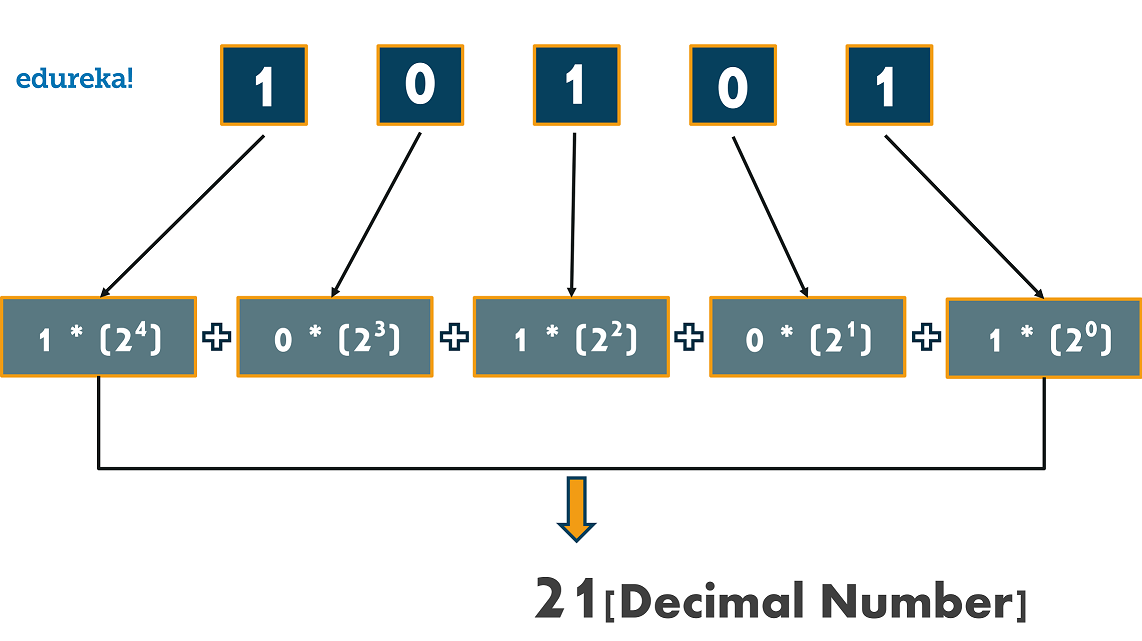# How To Convert Binary To Decimal In Java?20 / 29 Blog from Java Programs

Since the time, we have known that computers understand the binary language, there is no doubt in the fact that each one of us is inquisitive about converting the binary numbers to decimal, octal, and hexadecimal numbers. Well, in an environment where hundreds of numbers need to be decoded from machine language to a human interpreted language, it’s almost next to impossible to do it manually. So, instead, we can just write a simple code on how to convert binary to decimal in Java. Hence, in this article, I will discuss the same, in the following sequence:

Before I discuss the various ways of converting binary numbers to decimal numbers in Java, let us see the old school way of converting them.

## Mathematical conversion from Binary to Decimal

The idea is very simple. You just have to extract the digits of the binary number from the right-hand side and multiply it with a power of 2. Then, you have to add all the values to get the required decimal number. Refer to the below image:Since you have understood the mathematical conversion of binary to decimal numbers, let us understand how to write a code for it.

## Convert Binary to Decimal Numbers in Java

To convert a binary number to a decimal number in Java, you can use either the Integer.parseInt() method or custom logic. So, let us look into each one of them one by one. Starting with the Integer.parseInt() method:

### Interger.parseInt() method

This method is used to convert a string to an integer with the given radix. It is from the Integer class and the syntax of this method is as follows:

```public static int parseInt(String s,int radix)
```

#### Java Program using Integer.parseInt()

There are two ways in which you can write a Java program using Integer.parseInt(). The first way is to mention the binary number in the program itself, and the second way is to ask the user to enter the binary number.

##### Mention the binary number in the program itself
```package sampleprogram;
public class ConvertBinaryToDecimal {
public static void main(String args[]){
String binarynumber="10101";
int decimalnumber=Integer.parseInt(binarynumber,2);
System.out.println(decimalnumber);
}
}
```

Output:If you want to mention multiple binary numbers in the code itself, you can mention in the following way:

```package sampleprogram;
public class ConvertBinaryToDecimal {
public static void main(String args[]){
System.out.println(Integer.parseInt("1110",2));
System.out.println(Integer.parseInt("0010",2));
System.out.println(Integer.parseInt("1010",2));
System.out.println(Integer.parseInt("0110",2));
System.out.println(Integer.parseInt("1101",2));
}
}
```

Output:

#####Ask the user to enter the binary number

To make the user input the binary number, you have to import the Scanner class. The Scanner class is mainly used to get the user input, and it belongs to the java.util package.

```package sampleprogram;
import java.util.Scanner;
public class ConvertBinaryToDecimal {
public static void main(String args[]){
Scanner BinaryInput = new Scanner( System.in );
System.out.print("Enter the Binary Number - ");
String BinaryNumber =BinaryInput.nextLine();
System.out.println("Decimal Number- "+Integer.parseInt(BinaryNumber,2));
}
}
```

Output:Well, folks, that was about writing a java program using the Integer.parseInt() method. Now, next in this article on converting binary to decimal in java, let us see how to write a Java program for conversion of binary to decimal numbers without using the Integer.parseInt() method.

## Java program using custom logic

To write a Java program on how to convert a binary number to decimal number without using the Integer.parseInt() method, you can either write code by mentioning the binary numbers in the code itself or by taking the user input.

### Mention the binary number in the program itself

```package sampleprogram;
public class ConvertBinaryToDecimal {
public static int retrieveDecimal(int binarynumber){
int decimalnumber = 0;
int power = 0;
while(true)
{
if (binarynumber == 0)
{
break;
}
else
{
int temp = binarynumber%10;
decimalnumber += temp*Math.pow(2, power);
binarynumber = binarynumber/10;
power++;
}
}
return decimalnumber;
}
public static void main(String args[]){
System.out.println("Decimal value is: "+retrieveDecimal(1110));
System.out.println("Decimal value is: "+retrieveDecimal(0010));
System.out.println("Decimal value is: "+retrieveDecimal(1010));
System.out.println("Decimal value is: "+retrieveDecimal(0110));
System.out.println("Decimal value is: "+retrieveDecimal(1101));
}
}

```

###Ask the user to enter the binary number

```package sampleprogram;
import java.util.Scanner;
class ConvertBinaryToDecimal
{
public static void main(String args[])
{
Scanner binaryinput=new Scanner(System.in);
System.out.println("Enter the binary number-");
int n=binaryinput.nextInt();
int decimalnumber=0,power=0;
while(n!=0)
{
decimalnumber+=((n%10)*Math.pow(2,power));
n=n/10;
power++;
}
System.out.println(decimalnumber);
}
}
```
##### Output:This brings us to the end of this ‘How to Convert Binary to Decimal in Java?’ article. We have learned how to programmatically convert a binary number to a decimal number.

If you found this article on “How to Convert Binary to Decimal in Java?”, check out the Java Online Training by Edureka, a trusted online learning company with a network of more than 250,000 satisfied learners spread across the globe. We are here to help you with every step on your journey, for becoming a besides this java interview questions, we come up with a curriculum which is designed for students and professionals who want to be a Java Developer.

Got a question for us? Please mention it in the comments section of this “How to Convert Binary to Decimal in Java and we will get back to you as soon as possible.

Upcoming Batches For Java Certification Training Course
Course NameDate
Java Certification Training Course

Class Starts on 15th July,2023

15th July

SAT&SUN (Weekend Batch)
View DetailsREGISTER FOR FREE WEBINARThank you for registering Join Edureka Meetup community for 100+ Free Webinars each month